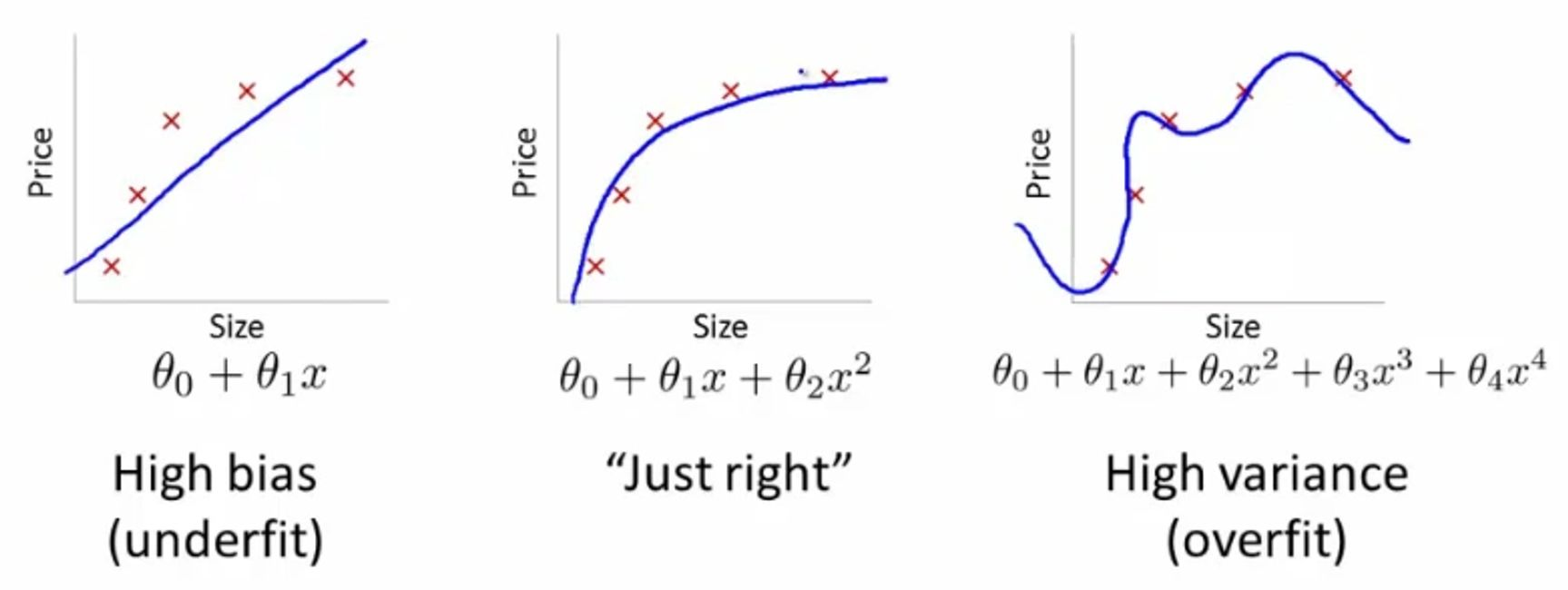Why We Don't Overfit

The Perennial Question

This is perhaps the first question asked of any data science methodology that tackles time series, and the one that is never resolved. The reason FMI does not encounter this problem is the following mathematical fact- for any given time series of length L and frequency f, there are a finite degrees of freedom that characterize the statistics of the system. No amount of feature engineering or neural network construction will get you to the perfect balance between overfitting and underfitting, where you are precisely capturing the information contained in the system and mod out all the noise. In fact our mathematicians have proved that FMI is the unique mathematical machinery to give you a models that sit precisely at this interface. In ML and especially neural networks, it is assumed that this balance can never be achieved, and hence there has to be some 'art' involved in the 'science'. FMI involves no art or redundancy and is thus immune to human bias. FMI captures all possible deviations from the efficient marke  hypothesis systematically.  That means that all possible market anomalies that are continuous in time are captured automatically. In addition we now also use unsupervised machine learning to capture discontinuous anomalies. In all, we provide you with a space of all possible trading strategies along with robustness estimates. This helps you create strategies with the risk-return combination you want.